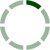### Posts by: Brendan K

Re: WLSRegression using Excel VBA
The function works using the range of cells in Sheet2; however, some of my beta values were extremely high.

They were: 6.8125
-0.1953125
0.000973804
-275
6976
-0.5625

Is it possible to have such large values? When you run the function, is this what you are getting? Thanks.
Go to post added 2 years ago
Re: WLSRegression using Excel VBA
Thanks. I plan to test this with the range of cells tonight.

As far as your question, OLSRegression will not work with an array because OLSRegression uses matrix multiplication?
Go to post added 2 years ago
Re: WLSRegression using Excel VBA
Actually, the 1's column is no longer needed - that was just used for the weights when my function was originally weighted least squares. All you need for PBS Betas, is the Implied Vol., the strike, and maturity. I removed the dimming of the variable types, and still getting the #Value. Maybe you... Read More
Go to post added 2 years ago
Re: WLSRegression using Excel VBA
I did modify OLSRegression as you mentioned, and made the final line OLSRegression = b, but I'm still getting the #VALUE error for the PBSBetas function output. Does modifying the OLSRegression also require any changes to the PBSBetas function?
Go to post added 2 years ago
Re: WLSRegression using Excel VBA
Here is some data to work with: Strike Maturity Implied Volatility 85 14 1.1300 95 14 0.6267 96 14 0.5783 98 14 0.5210 99 14 0.4899 99.5 14 0.4692 100 14 0.4613 101 14 0.4287 102 14 0.4012 103 14 0.3721 104 14 0.3413 105 14 0.3136 106 14 0.3081 107 14 0.2908 108 14 0.2634 109 14... Read More
Go to post added 2 years ago
Re: WLSRegression using Excel VBA
Yes, I do have some sample data. I will try to post by tomorrow. Thanks for the help.
Go to post added 2 years ago
Re: WLSRegression using Excel VBA
I modified the regression function for OLS, and determined it is working: Function OLSregression(y As Variant, X As Variant) As Variant Dim Xtrans As Variant, XX As Variant, XXinv As Variant, Xy As Variant Dim m1 As Variant, m2 As Variant, m3 As Variant Dim output() As Variant Xtrans = Applic... Read More
Go to post added 3 years ago
Re: WLSRegression using Excel VBA
Works perfectly. Thanks so much.
Go to post added 3 years ago
Re: WLSRegression using Excel VBA
I see. Is it normal to have a 0 in the first cell? Or, does it mean the function can be tweaked to run properly?
Go to post added 3 years ago
Re: WLSRegression using Excel VBA
Thanks. Yes, I finally got some actual data. For some reason though, the first cell is still 0. The other 2 are 22.14 and .01171. Is this what your output equaled? I did however, highlight 3 rows (down) instead of across the columns, since my code does include the transpose command as the last step ... Read More
Go to post added 3 years ago
 1 2 Next